# The Cardano family of equations

J. V. Leyendekkers and A. G. Shannon
Notes on Number Theory and Discrete Mathematics, ISSN 1310-5132
Volume 5, 1999, Number 4, Pages 151—162

## Details

### Authors and affiliations

J. V. Leyendekkers
The University of Sydney, 2006, Australia

A. G. Shannon
University of Technology, Sydney, 2007, Australia

### Abstract

The polynomial expansion of the Diophantine equation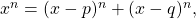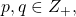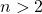, yields roots of the form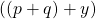where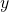is a non-integer zero of a Cardano cubic polynomial of the form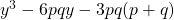. This is a corollary to Fermat’s Last Theorem. The characteristics of this family are illustrated for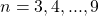. For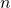odd,can be represented by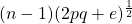, and foreven there are two real values of,and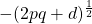, where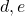are real non-integer parameters. For a given,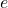is simply related to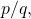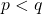, and to a parameter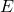which is linear in. The corresponding curves indicate the non-integral nature of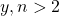.

• 11C08
• 11D41

### References

1. Boyer, Carl B. 1985. A History of Mathematics. Princeton: Princeton University Press.
2. Dunham, William. 1990. Journey through Genius: The Great Theorems of Mathematics. New York: John Wiley.
3. Galbraith, Steven. 1999. Elliptic Curve Public Key Cryptography. Mathematics Today’. 35(3): 76-79.
4. Gould, Henry’ W. 1999. The Girard — Waring Power Sum Formulas for Symmetric Functions and Fibonacci Sequences. The Fibonacci Quarterly. 37(2): 135-140.
5. Herz-Fischler, Roger. 1998. A Mathematical History of the Golden Section. New York: Dover.
6. Hillman, Abraham P. & Alexanderson, Gerald L. 1978. A First Undergraduate Course in Abstract Algebra. Second Edition. Belmont, CA: Wadsworth.
7. Householder, A.S. 1970. The Numerical Treatment of a Single Non-linear Equation. New York: McGraw-Hill.
8. McLeish, John. 1991. The Story of Numbers. New York: Fawcett Columbine.
9. Macmahon, Percy A. 1915. Combinatory Analysis. Volume I. Cambridge: Cambridge University Press.
10. de Pillis, L.G. 1998. Newton’s Cubic Roots. The Australian Mathematical Society Gazette. 25(5): 236-241.
11. Turnbull, H.W. 1952. Theory of Equations. Fifth Edition. Edinburgh: Oliver and Boyd.
12. van der Poorten, A. 1996. Notes on Fermat’s Last Theorem. New York: Wiley.

## Cite this paper

Leyendekkers, J. V. & Shannon, A. G. (1999). The Cardano family of equations. Notes on Number Theory and Discrete Mathematics, 5(4), 151-162.